# Lions Weekly: May 4, 2022# A Note From Mr. Sandefur

Lion Family,

Wow! What a week it has been on campus, and we are only halfway through this week. Our students are excelling in the classroom (from Pirate Phonics to dissections), in the arts (an incredible band concert and upcoming choir concert), and athletics (beginning tournaments and playoffs). One can get exhausted just looking at the schedule this time of year; however, the activity is exciting as it is encouraging to see our students diligently using the gifts and talents that the Lord has blessed them with. This week still holds more excitement, including Field Day (weather permitting), playoff soccer and baseball, the PTF Family Fun Fair, and so much more in our classrooms, chapels, etc. I want to invite you to any of our activities on campus so that you can celebrate this school year with us.

As we approach the finish line of this year, I would like to ask that you join me in praying for the class of 2022. As their days on campus continue to diminish, the legacy that this group leaves on campus indeed will not do the same. Please join me in praying as they finalize their next steps, celebrate their achievements, and cherish the special moments on the horizon. This group will always hold a special place in my heart as my first class of graduating seniors at TKA. Please join me in committing to pray for them and their families between now and graduation on May 20.

As always, it is an honor to partner with you and your family for your child’s educational journey as we seek to develop servant leaders that will change the world for Christ.

In Christ Alone,

Jeremy Sandefur
President

 table div table+table+table+table div table{width:100%;padding:0}table div table+table+table+table div table img{width:96.23%;padding:0;float:none}table div table+table+table+table div table td{width:100%;padding:0 1.88% 18px}/* styles */# Elementary Field Day

## Monday, May 9

Get ready for an awesome day of fun!

Due to forecasted rain, elementary field day has been rescheduled for Monday, May 9. K5-2nd will be from 8:30 a.m. to 11:00 a.m. and 3rd - 5th will be from 11:30 a.m to 2:00 p.m.

Thank you for your understanding as we look forward to a fun event with our Elementary Lions.

Theme: Survivor
When: K5-2nd 8:30am - 11:00am
3rd -5th 11:30am - 2:00pm
Where: Huskey Football field
Lunch: Hot dogs and hamburgers will be provided for students only by CNB bank.
What to bring: Sunscreen, towel, water bottle, & a change of clothes!

● Kindly, we ask for no pets at the event.
● Coach Robin is looking for volunteers! If you have interest in helping out on this awesome day, click here to sign up!

 table div table+table+table+table+table+table div table{width:100%;padding:0}table div table+table+table+table+table+table div table img{width:96.23%;padding:0;float:none}table div table+table+table+table+table+table div table td{width:100%;padding:0 1.88% 18px}/* styles */# PTF Family Fun Fair

PTF will host the Family Fun Fair on Saturday, May 7, from 10:00 a.m. to 2:00 p.m. This event will take place rain or shine. Join us for a day of fellowship and fun for all ages including inflatables, cakewalk, vendors, food truck and so much more!

 table div table+table+table+table+table+table+table+table div table{width:100%;padding:0}table div table+table+table+table+table+table+table+table div table img{width:96.23%;padding:0;float:none}table div table+table+table+table+table+table+table+table div table td{width:100%;padding:0 1.88% 18px}/* styles */# 2023 Washington, DC Trip

Next year March 2023 we will take Middle School students to Washington, DC.

This trip will include but is not limited to trips to :
- Arlington National Cemetery
- Mount Vernon
- Ford's Theatre / Petersen House
- Museum of the Bible
- Smithsonian Museums
- Holocaust Museum - Full visit
- United States Capitol
- United States Supreme Court
- Library of Congress
- White House Photo Stop
- Evening Illumination Tour of Memorials:
Vietnam, Korean, WWI, WWII & Iwo Jima Memorials,
Jefferson, FDR, MLK Jr., Eisenhower, & Lincoln Memorials,
Washington Monument - Photo Stop

Please join us on May 10th at 6:00 pm in the Woody Auditorium for a brief informational session where we will have more details regarding the trip including the registration process.

 table div table+table+table+table+table+table+table+table+table+table div table{width:100%;padding:0}table div table+table+table+table+table+table+table+table+table+table div table img{width:96.23%;padding:0;float:none}table div table+table+table+table+table+table+table+table+table+table div table td{width:100%;padding:0 1.88% 18px}/* styles */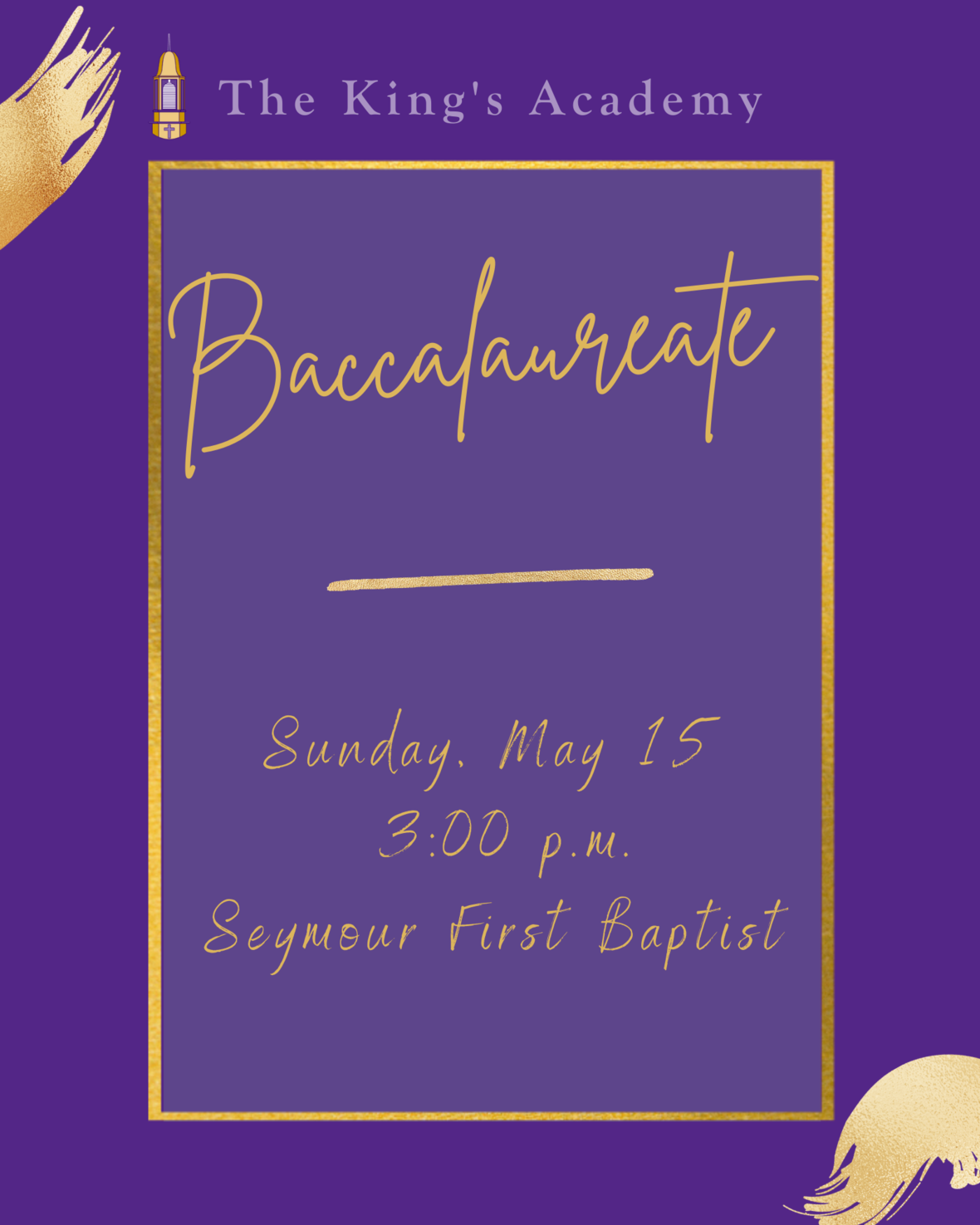# Baccalaureate Service

You are cordially invited to join us at Seymour First Baptist on Sunday, May 15, at 3:00 p.m. as we honor the graduating class of 2022. We will honor our students through words of encouragement, biblical challenges, a time of corporate worship, and a dedicated time of prayer and comissioning for our graduates.

 table div table+table+table+table+table+table+table+table+table+table+table+table div table{width:100%;padding:0}table div table+table+table+table+table+table+table+table+table+table+table+table div table img{width:96.23%;padding:0;float:none}table div table+table+table+table+table+table+table+table+table+table+table+table div table td{width:100%;padding:0 1.88% 18px}/* styles */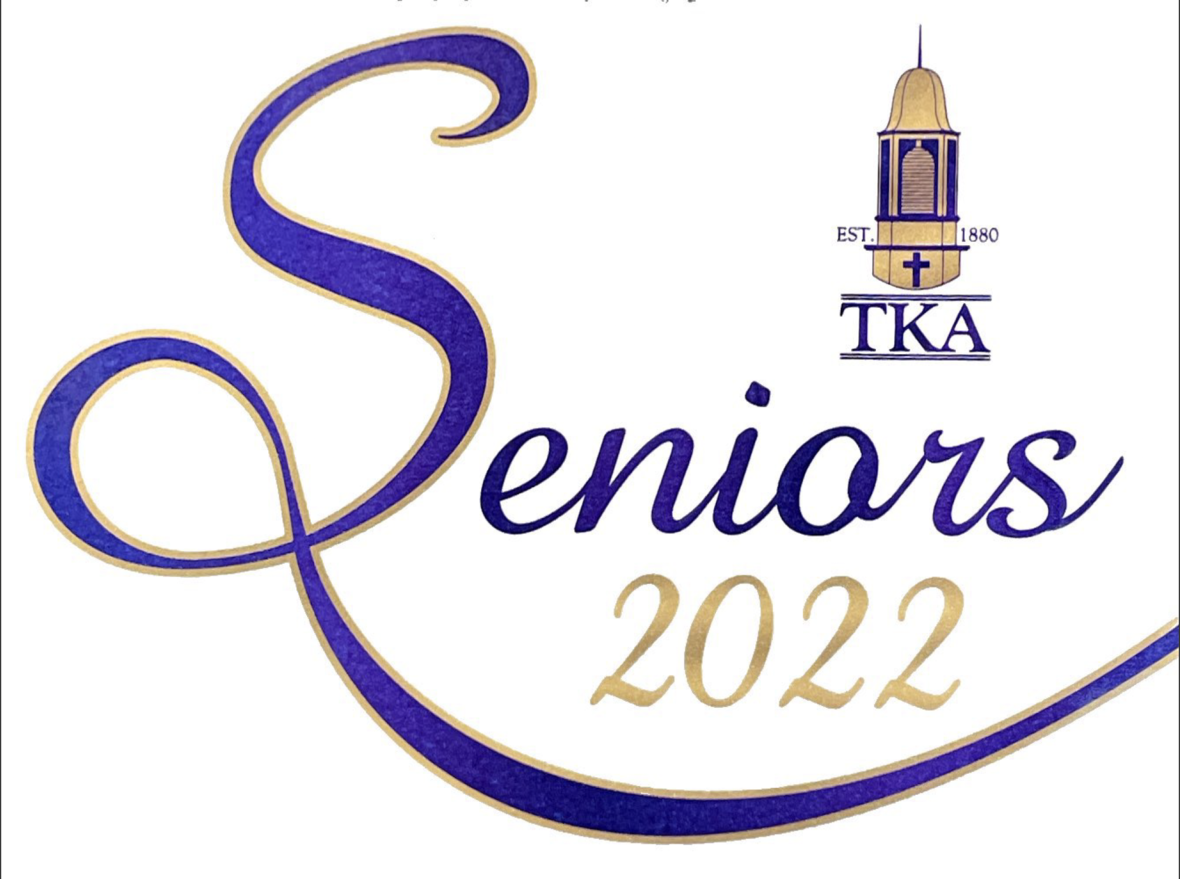On behalf of the faculty and graduating class of 2022, The King's Academy would like to invite you to the Commencement Exercises on Friday, May 20, at 7:00p.m. in Tibbetts.

 table div table+table+table+table+table+table+table+table+table+table+table+table+table+table div table{width:100%;padding:0}table div table+table+table+table+table+table+table+table+table+table+table+table+table+table div table img{width:96.23%;padding:0;float:none}table div table+table+table+table+table+table+table+table+table+table+table+table+table+table div table td{width:100%;padding:0 1.88% 18px}/* styles */# End of the Year Events

As the end of the year quickly approaches, we want to be sure and communicate any calendar events as soon as we are able. Below are upcoming events for the Month of May we would like for you to mark on your calendar.

Thursday, May 5
- Middle and High School Choir Concert at 6:30 p.m. in Woody Auditorium
Friday, May 6:
- Elementary Field Day from 8:30 a.m. to 2:00 p.m. (Huskey Field)
- Baseball Playoffs at TKA
Saturday, May 7:
- PTF Family Fun Fair from 10:00 a.m. to 2:00 p.m.
Tuesday, May 10:
- Middle School Washington, DC informational meeting at 6:00 p.m. in Woody Auditorium
Thursday, May 12:
- Elementary Spring Concert at 6:30 p.m. in Woody Auditorium
Friday, May 13:
- Senior Breakfast at 8:00 a.m.
- Legacy Walk at 9:00 a.m.
- Big/Little Buddy End of the Year Party at 10:30 a.m.
- Middle School Field Day from 1:00 p.m. to 3:00 p.m. at Tibbetts and the Baseball Field
- High School Field Day from 1:00p.m. to 3:00p.m. at Huskey Field and Stokley Gym
Sunday, May 15
- Baccalaureate Service at 3:00 p.m. at Seymour First Baptist
Tuesday, May 17
- Used Uniform Sale from 3:00 p.m. to 6:00 p.m. in Dr. Carroll Reece Reagan Pavilion
Thursday, May 19
- PTF Meeting at 3:15 in Brandes
Friday, May 20:
- High School Awards Day at 10:00 a.m. in Tibbetts
- Senior Graduation at 7:00 p.m. in Tibbetts
Monday, May 23:
- PreK4 Graduation at 6:00 p.m. in Woody Auditorium
Tuesday, May 24:
- HS Exams
- Sports Physicals at 3:00 p.m. in Tibbetts
- Kindergarten Graduation at 6:00 p.m. in Woody Auditorium
Wednesday, May 25
- HS Exams
Thursday, May 26:
- HS Exams
- 5th Grade promotion from 8:15 a.m. to 9:15 a.m. in Woody Auditorium
- Elementary classroom end of the year programs
Friday, May 27:
- Make-up HS Exams
- Middle School Awards at 9:00 a.m. in Woody Auditorium
- 8th Grade Promotion at 10:00 a.m. in Woody Auditorium
- Half-day dismissal at 11:30 a.m. No aftercare will be provided.

 table div table+table+table+table+table+table+table+table+table+table+table+table+table+table+table+table div table{width:100%;padding:0}table div table+table+table+table+table+table+table+table+table+table+table+table+table+table+table+table div table img{width:96.23%;padding:0;float:none}table div table+table+table+table+table+table+table+table+table+table+table+table+table+table+table+table div table td{width:100%;padding:0 1.88% 18px}/* styles */# Kenya Missions Fundraiser

Thank you to all who participated in our Kenya Missions Fundraiser! Through your generosity, we were able to offset a portion of the costs for our faculty/staff.

Please consider joining our effort to impact Empukani by supporting the cause with a separate donation. You can participate by clicking the link below.

We value your support of TKA’s mission to develop servant leaders who will change the world for Christ and for supporting our teachers in this effort!

Please join us in praying for our following team members as they prepare to leave in early June to provide VBS for students, teacher training, medical clinic assistance, and more: Lisa Quesinberry, Shannon Moore, Callie Smith, Jared Cartee, Julia Cartee, Lisa Sandefur, and Jeremy Sandefur.

 table div table+table+table+table+table+table+table+table+table+table+table+table+table+table+table+table+table+table+table div table{width:100%;padding:0}table div table+table+table+table+table+table+table+table+table+table+table+table+table+table+table+table+table+table+table div table img{width:96.23%;padding:0;float:none}table div table+table+table+table+table+table+table+table+table+table+table+table+table+table+table+table+table+table+table div table td{width:100%;padding:0 1.88% 18px}/* styles */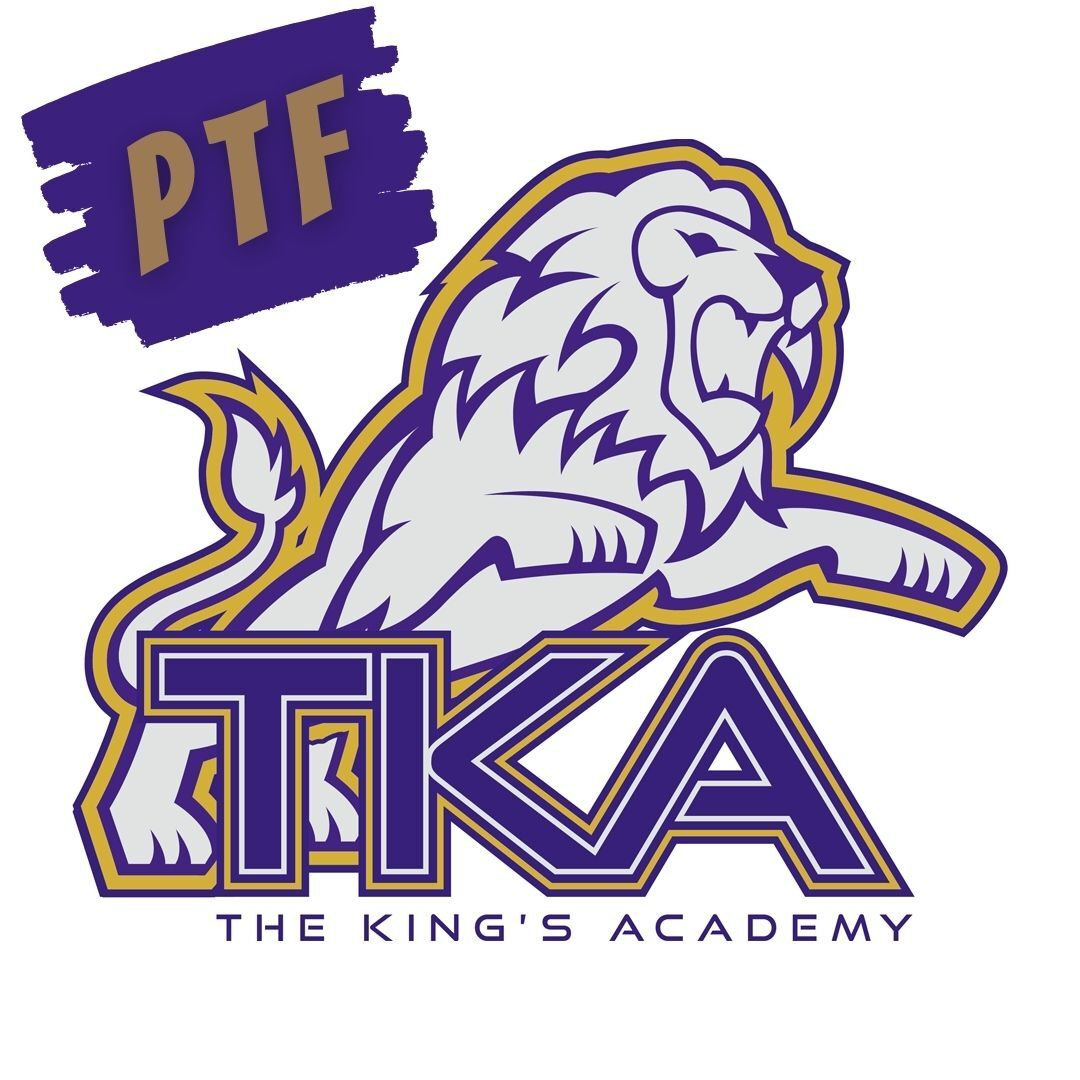# Save this Date!

Thursday, May 19, will be the last PTF meeting of the year in Brandes from 3:15 - 4:15. All members are encouraged to attend.

 table div table+table+table+table+table+table+table+table+table+table+table+table+table+table+table+table+table+table+table+table+table div table{width:100%;padding:0}table div table+table+table+table+table+table+table+table+table+table+table+table+table+table+table+table+table+table+table+table+table div table img{width:96.23%;padding:0;float:none}table div table+table+table+table+table+table+table+table+table+table+table+table+table+table+table+table+table+table+table+table+table div table td{width:100%;padding:0 1.88% 18px}/* styles */# Used Uniform Sale

When: Tuesday, May 17, from 3pm-6pm
What: Bring all your outgrown, new or used polos, pants/shorts/skirts, spirit wear, shoes, backpacks/bags & ANYTHING TKA-related. Don’t forget about Daddy-Daughter & Prom dresses/attire. And sport equipment…. If TKA plays it, bring it!

Where: We will set up a “uniform shop” under the Dr. Carroll Reece Reagan Pavilion so you can sell your own items for whatever price you choose.

Hopefully we can reuse and repurpose and bless each other. Stay as little or as long as you wish!

If it is not allowed in the dress code, please do not bring it (remember that pink & light blue are not allowed starting next school year)

 table div table+table+table+table+table+table+table+table+table+table+table+table+table+table+table+table+table+table+table+table+table+table+table div table{width:100%;padding:0}table div table+table+table+table+table+table+table+table+table+table+table+table+table+table+table+table+table+table+table+table+table+table+table div table img{width:96.23%;padding:0;float:none}table div table+table+table+table+table+table+table+table+table+table+table+table+table+table+table+table+table+table+table+table+table+table+table div table td{width:100%;padding:0 1.88% 18px}/* styles */# 27th Annual Golf Tournament

Join us at Egwani Farms on June 13, for our 27th Annual Golf Tournament to benefit TKA's football team!

Gather your company, friends or family and purchase one of the following sponsorships:
- Purple Sponsor includes a 4-person team and a hole sponsorship for \$500
- Silver Sponsor includes an individual player and a hole sponsorship for \$200
- Individual players can participate for \$100

 table div table+table+table+table+table+table+table+table+table+table+table+table+table+table+table+table+table+table+table+table+table+table+table+table+table div table{width:100%;padding:0}table div table+table+table+table+table+table+table+table+table+table+table+table+table+table+table+table+table+table+table+table+table+table+table+table+table div table img{width:96.23%;padding:0;float:none}table div table+table+table+table+table+table+table+table+table+table+table+table+table+table+table+table+table+table+table+table+table+table+table+table+table div table td{width:100%;padding:0 1.88% 18px}/* styles */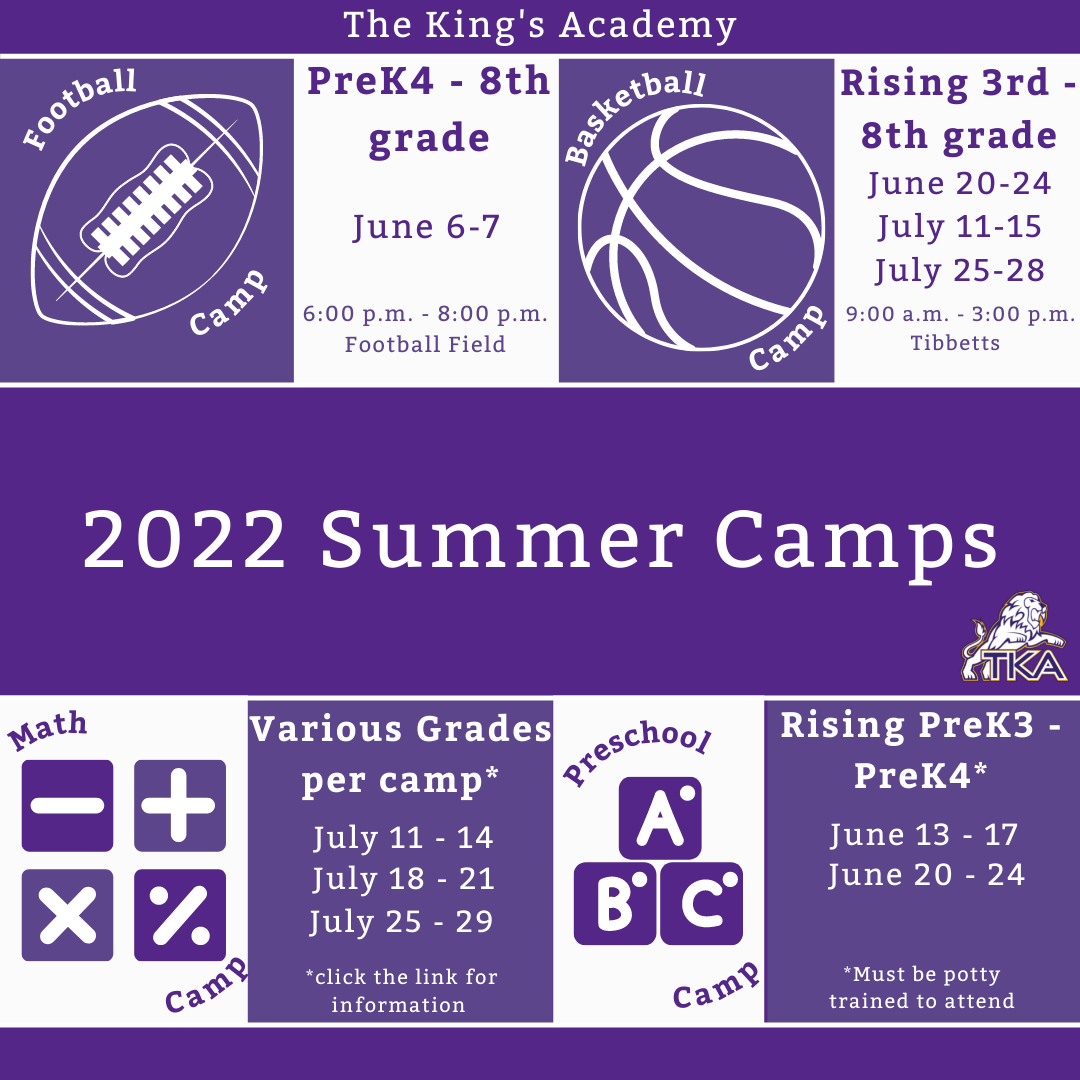# 2022 Summer Camps

We are excited to offer a variety of camps for our TKA Lions this summer! Each camp will be listed below with a link to register for each event. We are looking forward to seeing our Lions on campus at these fun camps!

 table div table+table+table+table+table+table+table+table+table+table+table+table+table+table+table+table+table+table+table+table+table+table+table+table+table+table+table div table{width:100%;padding:0}table div table+table+table+table+table+table+table+table+table+table+table+table+table+table+table+table+table+table+table+table+table+table+table+table+table+table+table div table img{width:96.23%;padding:0;float:none}table div table+table+table+table+table+table+table+table+table+table+table+table+table+table+table+table+table+table+table+table+table+table+table+table+table+table+table div table td{width:100%;padding:0 1.88% 18px}/* styles */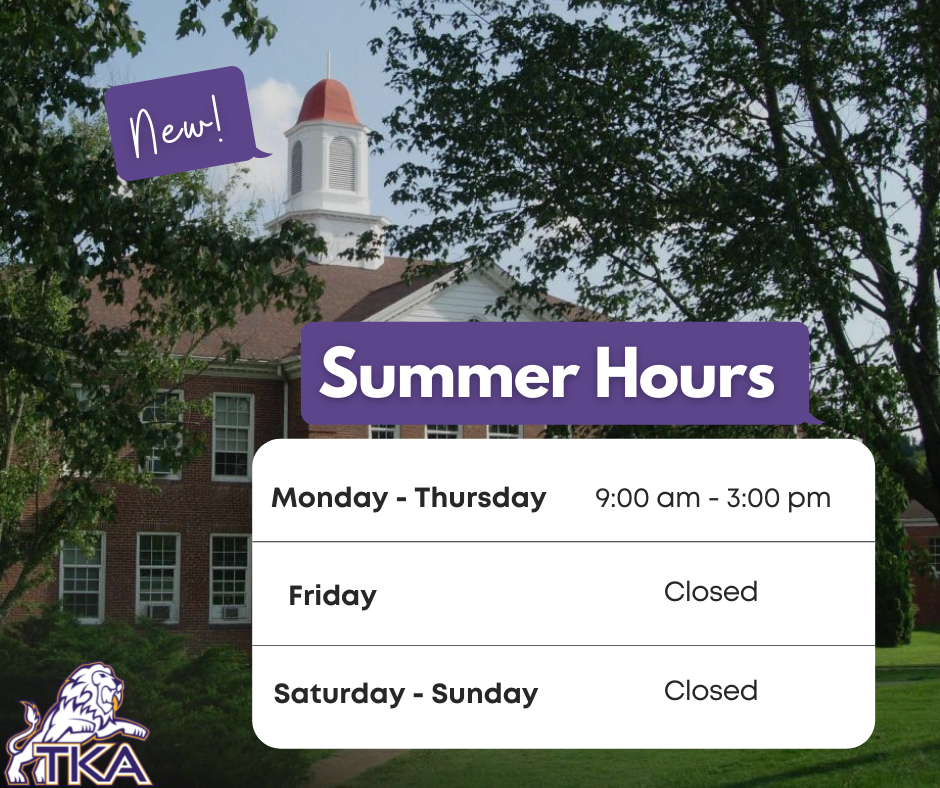# Summer 2022

This summer the school offices (Anderson and Ogle) will be open Monday - Thursday from 9:00 a.m. to 3:00 p.m. The offices will be closed Friday through Sunday.

 table div table+table+table+table+table+table+table+table+table+table+table+table+table+table+table+table+table+table+table+table+table+table+table+table+table+table+table+table+table div table{width:100%;padding:0}table div table+table+table+table+table+table+table+table+table+table+table+table+table+table+table+table+table+table+table+table+table+table+table+table+table+table+table+table+table div table img{width:96.23%;padding:0;float:none}table div table+table+table+table+table+table+table+table+table+table+table+table+table+table+table+table+table+table+table+table+table+table+table+table+table+table+table+table+table div table td{width:100%;padding:0 1.88% 18px}/* styles */# Spring Sport Highlights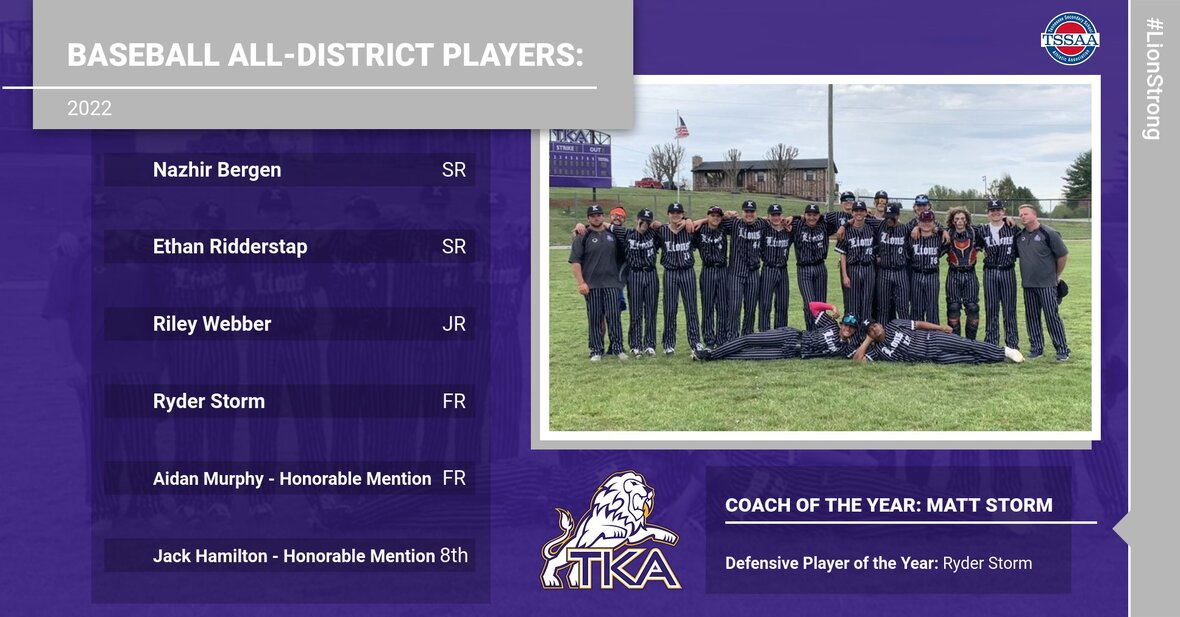# Varsity Baseball

TKA baseball is back at it again with more exciting news. Congratulations to our All-District baseball players: Nazhir Bergen, Ethan Ridderstap, Riley Webber, and Ryder Storm! Congratulations Aidan Murphy and Jack Hamilton for being named honorable mentions in the district! Ryder Storm was also named Defensive player of the year, and Head Coach, Matt Storm, was named Coach of the Year! We are proud of all of our Lions!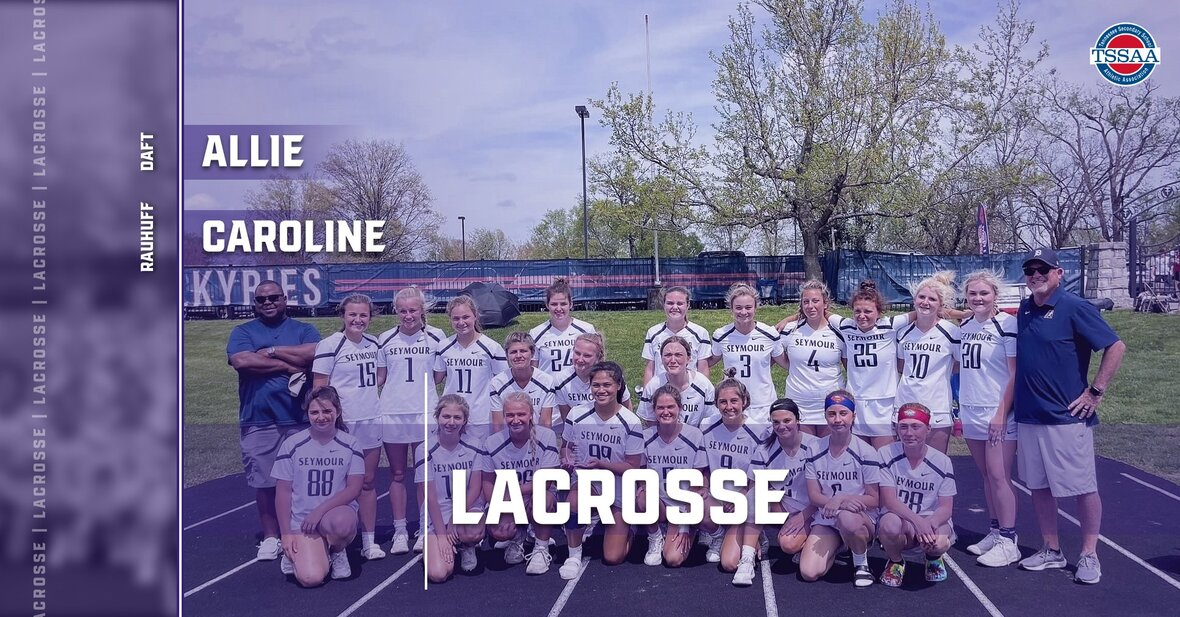# Lacrosse

It’s time for another spring sport highlight! Allie Daft and Caroline Rauhuff have been playing Lacrosse with our Co-op partners at Seymour High School. Caroline was chosen as defensive All-Region, and they will be playing this weekend in the final four games! We are proud of how these ladies have represented TKA on and off the field!

 table div table+table+table+table+table+table+table+table+table+table+table+table+table+table+table+table+table+table+table+table+table+table+table+table+table+table+table+table+table+table+table+table+table div table{width:100%;padding:0}table div table+table+table+table+table+table+table+table+table+table+table+table+table+table+table+table+table+table+table+table+table+table+table+table+table+table+table+table+table+table+table+table+table div table img{width:96.23%;padding:0;float:none}table div table+table+table+table+table+table+table+table+table+table+table+table+table+table+table+table+table+table+table+table+table+table+table+table+table+table+table+table+table+table+table+table+table div table td{width:100%;padding:0 1.88% 18px}/* styles */# Upcoming Sports# Varsity Soccer

## Thursday, May 5, at 4:00p.m.

TKA will host the second round of the TSSAA soccer playoffs at Johnson University on Thursday, May 5, at 4:00p.m. The cost is \$5.00 per person at the gate. Come out and cheer on our Lions!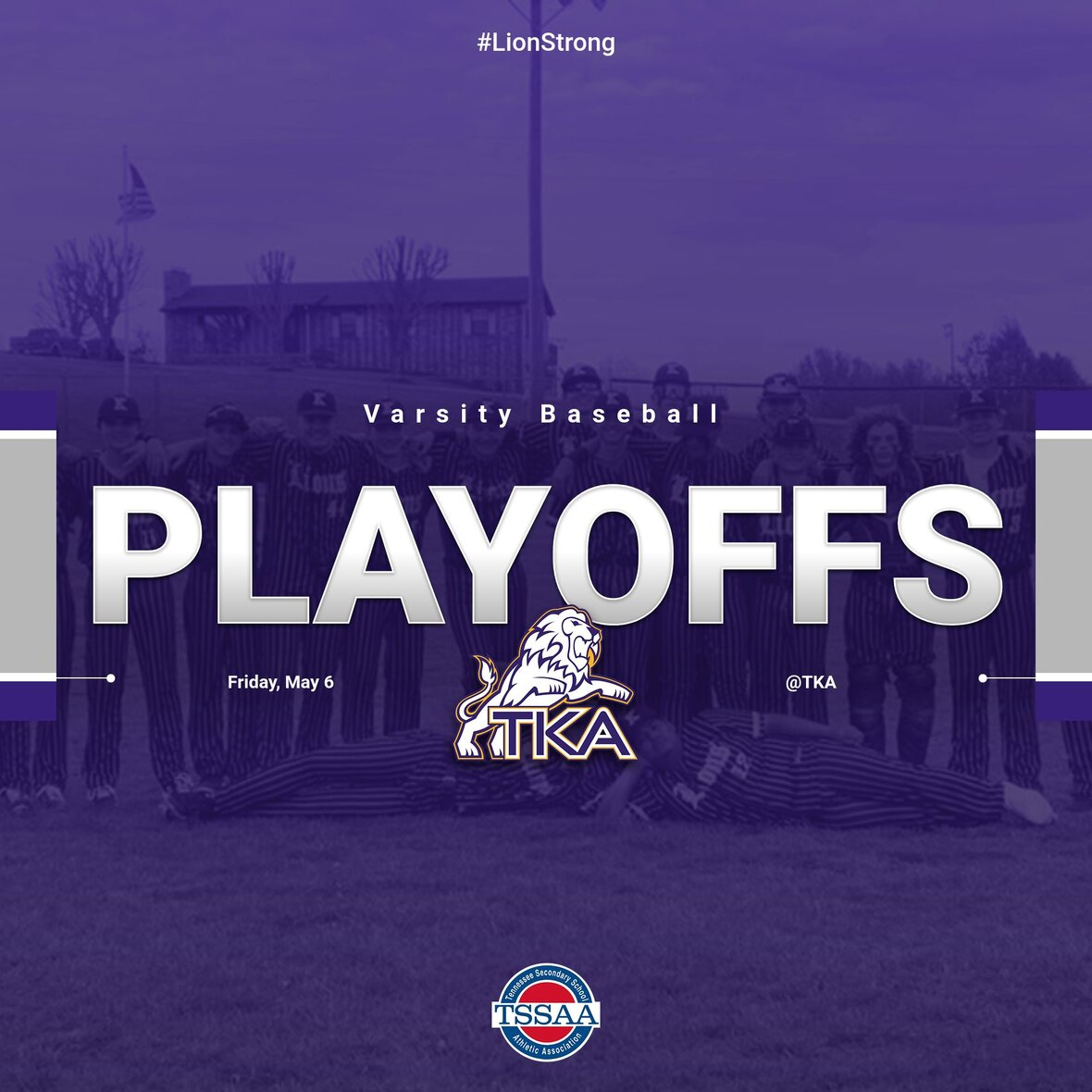# Varsity Baseball

## Friday, May 6, at 4:00p.m. and 6:00p.m.

Mark your calendars! Friday, May 6, TKA will host the first round of the TSSAA Baseball playoffs. Our Lions will host Boyd Buchanan School at 4:00p.m. and 6:00p.m. Game three will be held on Saturday, May 7 if needed. The cost is \$5.00 per person, per day, at the gate. We look forward to seeing you at the games!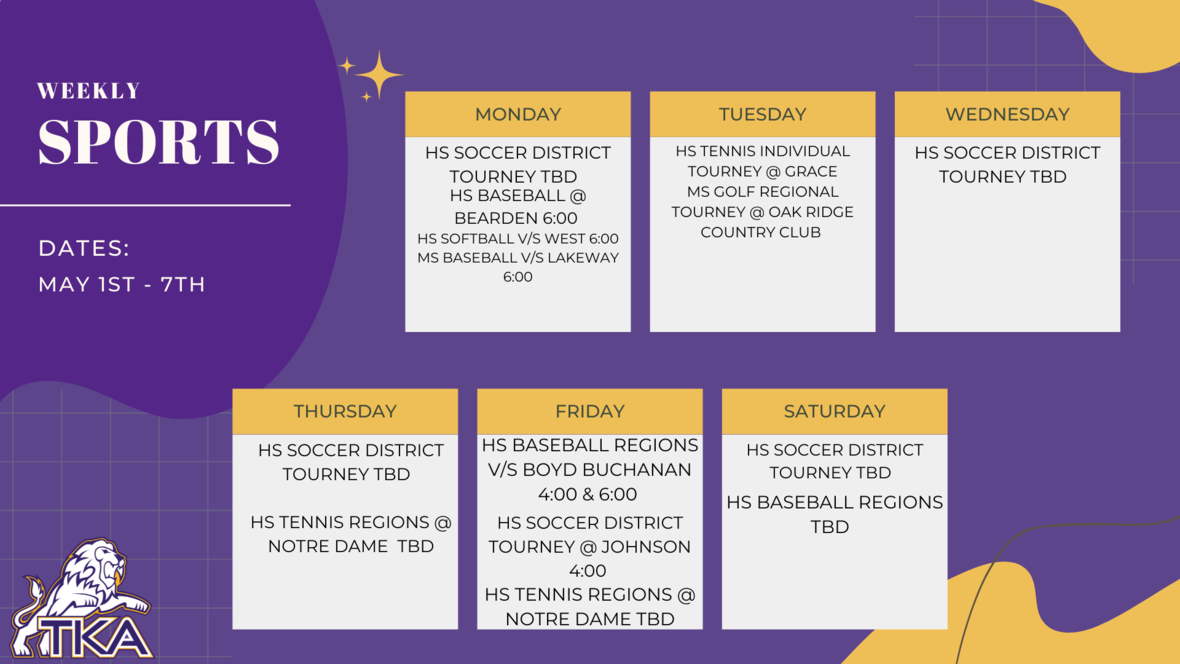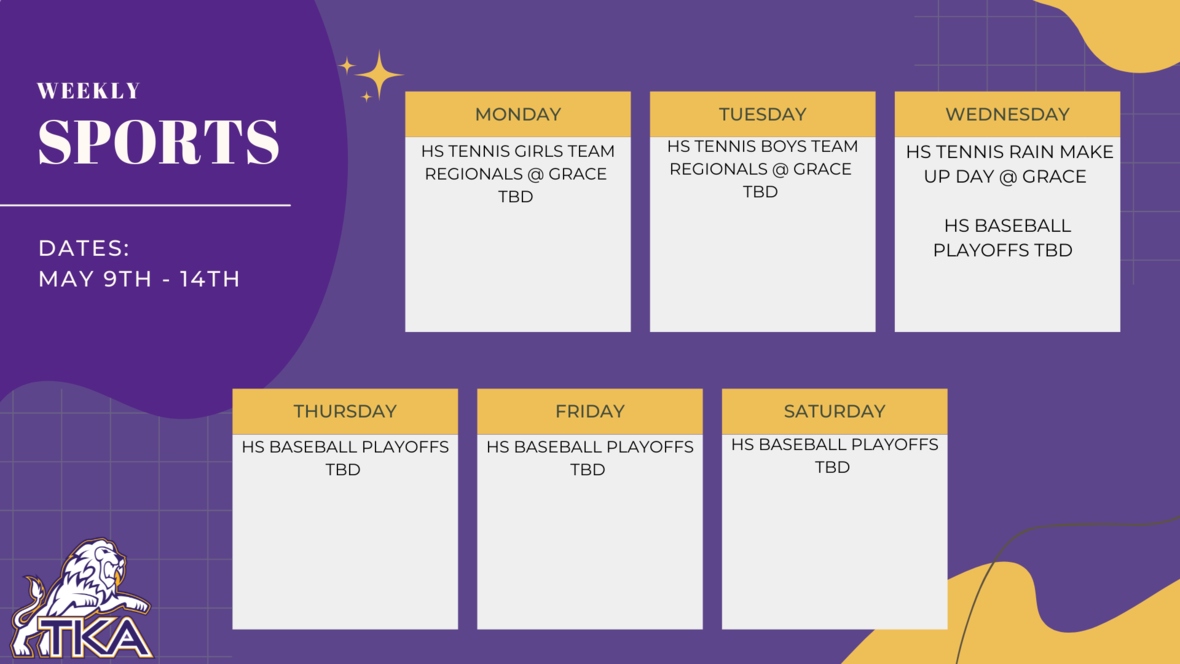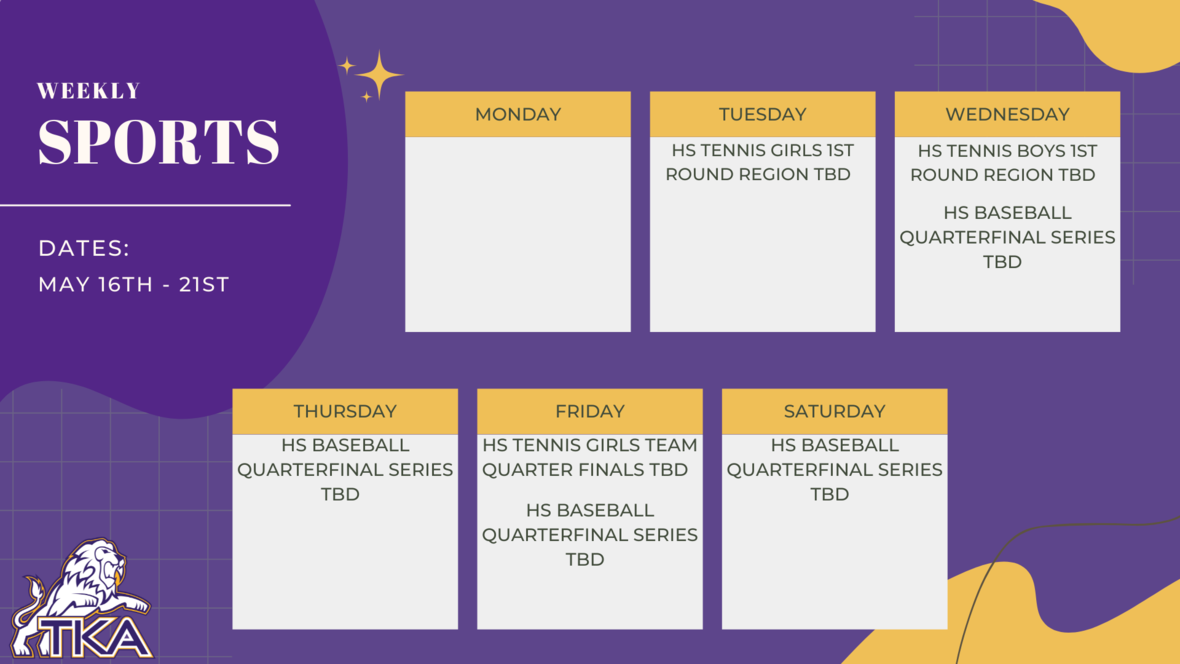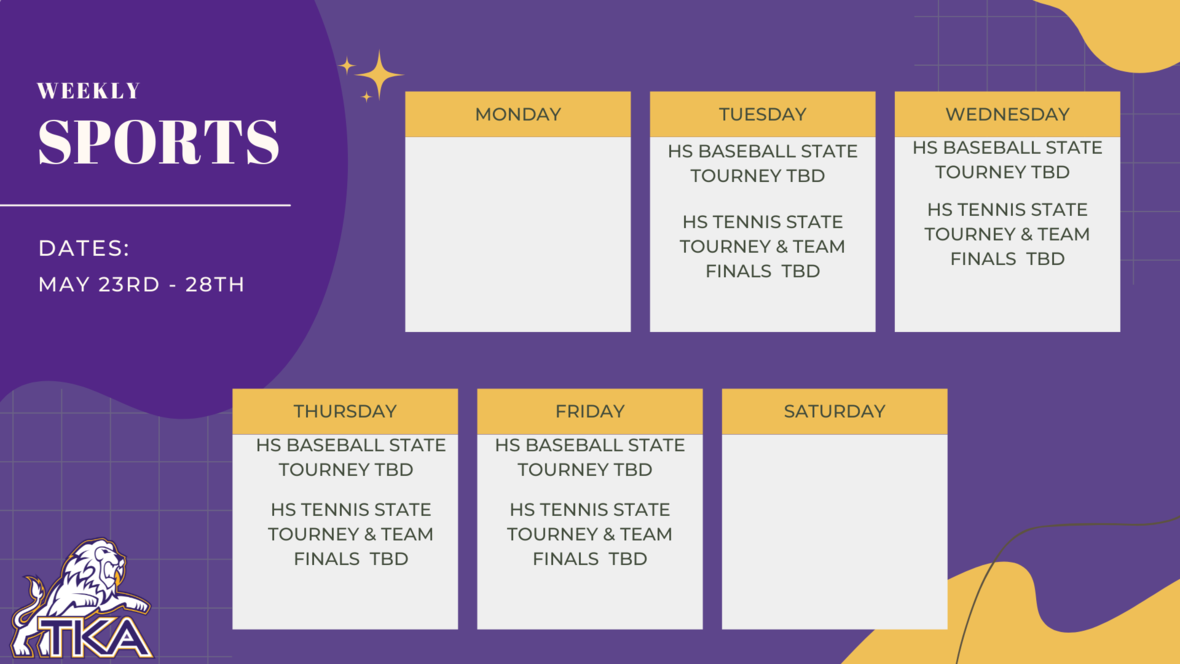table div table+table+table+table+table+table+table+table+table+table+table+table+table+table+table+table+table+table+table+table+table+table+table+table+table+table+table+table+table+table+table+table+table+table+table+table+table+table+table+table+table div table{width:100%;padding:0}table div table+table+table+table+table+table+table+table+table+table+table+table+table+table+table+table+table+table+table+table+table+table+table+table+table+table+table+table+table+table+table+table+table+table+table+table+table+table+table+table+table div table img{width:96.23%;padding:0;float:none}table div table+table+table+table+table+table+table+table+table+table+table+table+table+table+table+table+table+table+table+table+table+table+table+table+table+table+table+table+table+table+table+table+table+table+table+table+table+table+table+table+table div table td{width:100%;padding:0 1.88% 18px}/* styles */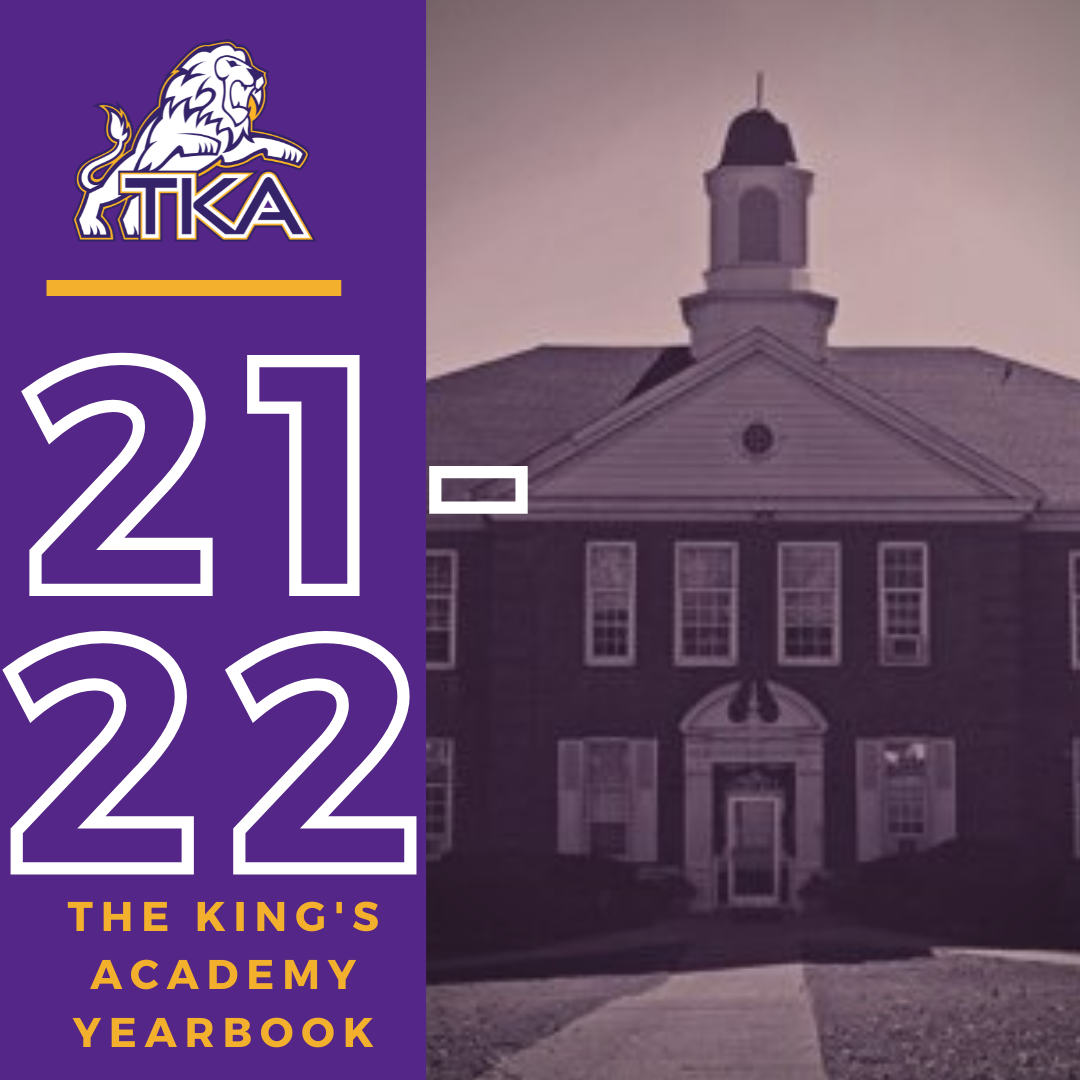# 2021-2022 Yearbook

The price for the 2021-2022 TKA yearbook is \$55. All grades, K3-12 will have the same yearbook. The slight price increase is because we are only going to have one larger all-school book.

All yearbook orders can be placed online--here is the link.Do you have a class of 2022 senior in your household?

Don't miss out on the opportunity to purchase a Senior Tribute for the 2021-2022 yearbook!

Senior Tributes are available for purchase at the bottom of the link listed here.For any questions regarding yearbooks, email Mr. Josh Smith at jsmith@thekingsacademy.net.

 table div table+table+table+table+table+table+table+table+table+table+table+table+table+table+table+table+table+table+table+table+table+table+table+table+table+table+table+table+table+table+table+table+table+table+table+table+table+table+table+table+table+table+table+table+table div table{width:100%;padding:0}table div table+table+table+table+table+table+table+table+table+table+table+table+table+table+table+table+table+table+table+table+table+table+table+table+table+table+table+table+table+table+table+table+table+table+table+table+table+table+table+table+table+table+table+table+table div table img{width:96.23%;padding:0;float:none}table div table+table+table+table+table+table+table+table+table+table+table+table+table+table+table+table+table+table+table+table+table+table+table+table+table+table+table+table+table+table+table+table+table+table+table+table+table+table+table+table+table+table+table+table+table div table td{width:100%;padding:0 1.88% 18px}/* styles */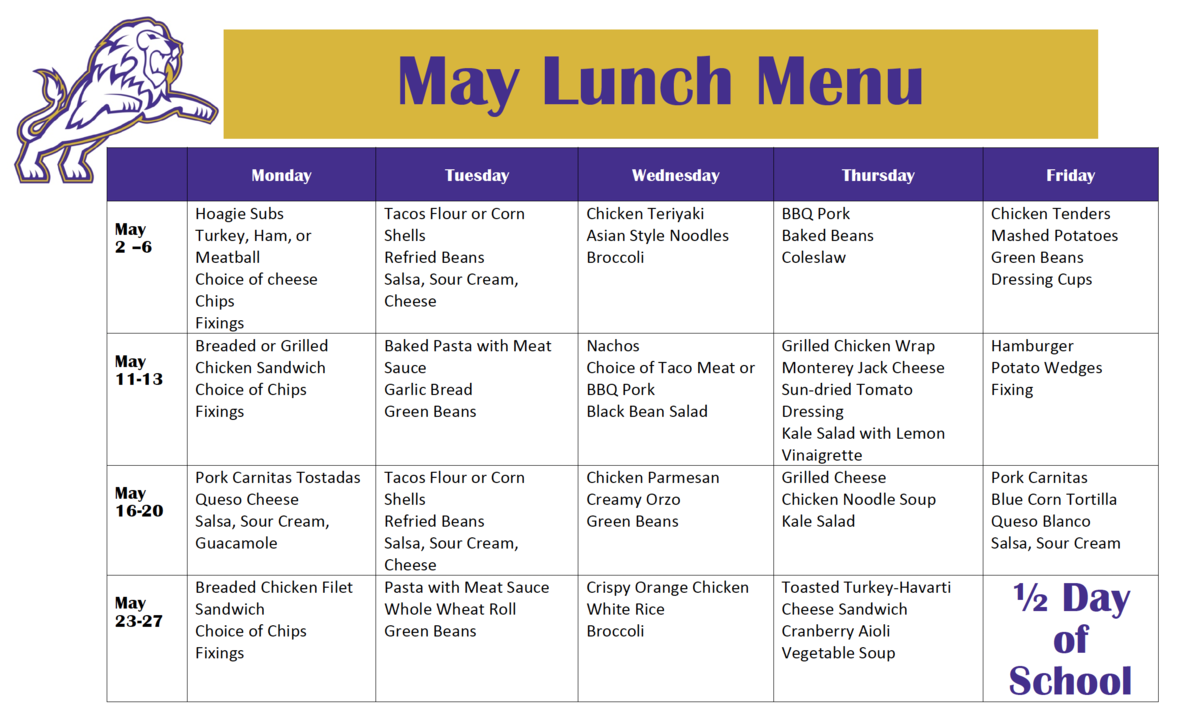table div table+table+table+table+table+table+table+table+table+table+table+table+table+table+table+table+table+table+table+table+table+table+table+table+table+table+table+table+table+table+table+table+table+table+table+table+table+table+table+table+table+table+table+table+table+table+table div table{width:100%;padding:0}table div table+table+table+table+table+table+table+table+table+table+table+table+table+table+table+table+table+table+table+table+table+table+table+table+table+table+table+table+table+table+table+table+table+table+table+table+table+table+table+table+table+table+table+table+table+table+table div table img{width:96.23%;padding:0;float:none}table div table+table+table+table+table+table+table+table+table+table+table+table+table+table+table+table+table+table+table+table+table+table+table+table+table+table+table+table+table+table+table+table+table+table+table+table+table+table+table+table+table+table+table+table+table+table+table div table td{width:100%;padding:0 1.88% 18px}/* styles *//* styles */
 table div table+table+table+table+table+table+table+table+table+table+table+table+table+table+table+table+table+table+table+table+table+table+table+table+table+table+table+table+table+table+table+table+table+table+table+table+table+table+table+table+table+table+table+table+table+table+table+table+table+table div table{width:100%;padding:0}table div table+table+table+table+table+table+table+table+table+table+table+table+table+table+table+table+table+table+table+table+table+table+table+table+table+table+table+table+table+table+table+table+table+table+table+table+table+table+table+table+table+table+table+table+table+table+table+table+table+table div table img{width:96.23%;padding:0;float:none}table div table+table+table+table+table+table+table+table+table+table+table+table+table+table+table+table+table+table+table+table+table+table+table+table+table+table+table+table+table+table+table+table+table+table+table+table+table+table+table+table+table+table+table+table+table+table+table+table+table+table div table td{width:100%;padding:0 1.88% 18px}/* styles */# Covid-19 Procedures

While we are excited to offer in-person education, we must maintain a safe learning environment for all students, faculty/staff, and additional stakeholders.

To do so, we will be working closely with the Sevier County Health Department regarding Covid-19 protocols.

 table div table+table+table+table+table+table+table+table+table+table+table+table+table+table+table+table+table+table+table+table+table+table+table+table+table+table+table+table+table+table+table+table+table+table+table+table+table+table+table+table+table+table+table+table+table+table+table+table+table+table+table+table div table{width:100%;padding:0}table div table+table+table+table+table+table+table+table+table+table+table+table+table+table+table+table+table+table+table+table+table+table+table+table+table+table+table+table+table+table+table+table+table+table+table+table+table+table+table+table+table+table+table+table+table+table+table+table+table+table+table+table div table img{width:96.23%;padding:0;float:none}table div table+table+table+table+table+table+table+table+table+table+table+table+table+table+table+table+table+table+table+table+table+table+table+table+table+table+table+table+table+table+table+table+table+table+table+table+table+table+table+table+table+table+table+table+table+table+table+table+table+table+table+table div table td{width:100%;padding:0 1.88% 18px}/* styles */table div table+table+table+table+table+table+table+table+table+table+table+table+table+table+table+table+table+table+table+table+table+table+table+table+table+table+table+table+table+table+table+table+table+table+table+table+table+table+table+table+table+table+table+table+table+table+table+table+table+table+table+table+table+table div table{width:100%;padding:0}table div table+table+table+table+table+table+table+table+table+table+table+table+table+table+table+table+table+table+table+table+table+table+table+table+table+table+table+table+table+table+table+table+table+table+table+table+table+table+table+table+table+table+table+table+table+table+table+table+table+table+table+table+table+table div table img{width:96.23%;padding:0;float:none}table div table+table+table+table+table+table+table+table+table+table+table+table+table+table+table+table+table+table+table+table+table+table+table+table+table+table+table+table+table+table+table+table+table+table+table+table+table+table+table+table+table+table+table+table+table+table+table+table+table+table+table+table+table+table div table td{width:100%;padding:0 1.88% 18px}/* styles */